# Abstract

This paper describes an extended formulation for the coupled beam method (CBM). The method is originally developed for elastic bending response analysis of passenger ships with large multi-deck superstructures. The extension is mainly performed to enable the available method in order to study elastic bending behaviour of ships fitted with superstructures of any sizes and locations. Finite element method (FEM) is applied for solving the equilibrium equations. Both hull and superstructure of the ship are modelled using beam elements. The connection between beam elements representing hull and superstructure is made using specially developed spring box elements. The accuracy of the extended method is demonstrated using an available experimental result. Then, two simplified structures, one representing a ship with a short superstructure and the other one representing a ship with a long superstructure, are analysed in order to validate the extended coupled beam method against the finite element method. In spite of some existing simplifications in the extended formulation, it is very effective in the early stages of ship structural design owing to its advantageous capability of rapid estimation of the longitudinal stress distributions along the height of ships at different stations.

hull; superstructure; interaction; bending; Coupled Beam Method (CBM); Finite Element Method (FEM)

An extension of coupled beam method and its application to study ship's hull-superstructure interaction problems

Fattaneh MorshedsoloukI; Mohammad Reza KhedmatiII,* * Author email: khedmati@aut.ac.ir

IFaculty of Marine Technology, Amirkabir University of Technology, Tehran 15914  Iran

IIFaculty of Marine Technology, Amirkabir University of Technology, Tehran 15914  Iran

ABSTRACT

This paper describes an extended formulation for the coupled beam method (CBM). The method is originally developed for elastic bending response analysis of passenger ships with large multi-deck superstructures. The extension is mainly performed to enable the available method in order to study elastic bending behaviour of ships fitted with superstructures of any sizes and locations. Finite element method (FEM) is applied for solving the equilibrium equations. Both hull and superstructure of the ship are modelled using beam elements. The connection between beam elements representing hull and superstructure is made using specially developed spring box elements. The accuracy of the extended method is demonstrated using an available experimental result. Then, two simplified structures, one representing a ship with a short superstructure and the other one representing a ship with a long superstructure, are analysed in order to validate the extended coupled beam method against the finite element method. In spite of some existing simplifications in the extended formulation, it is very effective in the early stages of ship structural design owing to its advantageous capability of rapid estimation of the longitudinal stress distributions along the height of ships at different stations.

Keywords: hull, superstructure, interaction, bending, Coupled Beam Method (CBM), Finite Element Method (FEM)

1 INTRODUCTION

Size and location of superstructures fitted on the deck of ship's hull structures depend on the ship type. Typical cargo ships have generally short superstructures, which may be fitted at any locations along the ship. This is while, long and wide superstructures are found on large passenger ships. The extent of a superstructure's contribution to the overall strength of a ship has been a matter of interest to many researchers and also has been debated for many years. Figure 1 summarises the history of key methods and studies applied in investigation

NOTATION

Ai cross-sectional area of the i-th beamnodal cross-sectional area of the i-th beam Cij bending moment lever on the i-th beam due to the shearing force between the i-th and j-th beams d ik distance between the upper fibre of the beam to the reference line eij distance between the lower fibre of the beam to the reference line E Modulus of elasticity F external nodal forces matrix Hij height of shear element between i-th and j-th beams Ii sectional moment of inertia of the i-th beam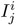nodal sectional moment of inertia of the i-th beam K global stiffness matrix of system Ki stiffness matrix of i-th beam kij transverse stiffness between the i-th and j-th beams KShear stiffness matrix for the shearing springs KTrans stiffness matrix for the transverse springs Mi bending moment of the i-th beam n number of beams Ni axial force of the i-th beam Nj(x) shape functions pij transverse (vertical) distributed forces between the i-th and j-th beams Qi shear force of the i-th beam qi external force of the i-th beam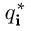approximate function for external force of the i-th beam sij longitudinal distributed shear forces between the i-th and j-th beamsapproximate function for longitudinal distributed shear forces between the i-th and j-th beams Tij shear stiffness between the i-th and j-th beams ui axial displacement of the i-th beamapproximate solution to axial displacement for the i-th beam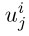the j-th node of the i-th beam axial displacement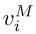transverse deflection of the i-th beam caused by bendingapproximate solution to transverse displacement for the i-th beam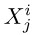the j-th node of the i-th beam transverse displacement X matrix of the degrees of freedom for the i-th beam Xi first sectional moment of area of the i-th beamnodal first sectional moment of area of the i-th beam Xs nodal displacement vector of systemthe relative displacement between beam i and beam jthe j-th node of the i-th beam angular displacement

of superstructure effects on the longitudinal bending strength of ship's hull structures. An excellent literature survey until 1983 is given by de Oliveira .

Bruhn  seems to have been the first to study in detail the influence of discontinuities on the stress distribution in ships. He recognised the importance of superstructure length on the stress distributions above the main deck. The studies made by Bruhn were based upon tests on sheet rubber models. King  examined the effect on longitudinal stresses of adding material at various heights above the hull girder in single and multiple tiers in an attempt to rationalise the scantlings of large superstructures. The results of King  showed the possibility of designing a superstructure capable of participating fully in the ship's longitudinal strength provided adequate transitions at both ends of superstructure were fitted. Hovgaard [13, 14] was probably the first to fully recognise the important role played by shear stresses at the hull-superstructure connection. He developed a theory of shear stresses in riveted and welded joints and applied it to discontinuities that occur in ship structures. Vasta [25, 26] seems to have been the first to use a measure of effectiveness to characterise the superstructure behaviour.

Analytical methods are categorised into two basic approaches, based on either two-beam theory or plane stress theory. Crawford  was the first to develop the method based on the two-beam theory, taking into account the longitudinal shear force and the vertical force due to the hull-superstructure interaction. Bleich's approach  is similar to that of Crawford, but describing a straightforward procedure for computation of normal stresses for prismatic beams. Chapman  presented a method for finding the deflections and stresses along the length of a ship, taking into account of distortion of the hull cross-section as a result of its interaction with the superstructure. Terazawa and Yagi  introduced the shear lag correction to the two-beam theory. The stresses were calculated using the energy approach and assuming predefined stress patterns for the structure. They also considered the effect of side openings on the structural behaviour. Simplified approach to generate global response of large catamarans with the large superstructure windows at the early stages of design using extended beam theory was presented by Heggelund and Moan . Naar et al.  proposed a new approach called coupled beams method (CBM) to evaluate hull girder response of passenger ships. This method is based on the assumption that the global bending response of a modern passenger ship can be estimated by help of beams coupled to each other by distributed longitudinal and vertical springs.

Caldwell  presented a method based on plane stress theory in order to determine how the superstructure efficiency in hull bending strength varies with the ratio of its length to transverse dimensions, with the flexibility of the upper deck and with the distribution of bending moments applied on the ship. Johnson  also developed plane stress theory to study the stresses in deckhouses and superstructures. In his approach, the shear stress distribution along the edges of the deckhouse sides was assumed to be linear. The theory could take care of the possibility of deckhouse having several decks. Fransman  developed methods based on the plane stress theory and made an improvement with respect to Caldwell  approach.

Also some research studies on the topic of hull-superstructure interaction were made using the finite element method (FEM). Paulling and Payer  and Mitchell  have obtained stresses and deflections using the finite element method and compared their results with separate experimental results of rectangular hull-deckhouse models. The work of McVee  follows with the implementation to naval ship problems where the hulldeckhouse interaction is one of the most important structural issues. In Zanic and Jancijev  a somewhat simpler combination of 2D finite element membrane model of ship projected onto the centreline plane and the partial 3D finite element model around midship section was applied to simulate the global structural response of night ferry and to perform structural optimisation of the amidships part. Andreau and Gillet  give a short overview of dominant structural parameters that influenced longitudinal strength of passenger ships with the extensive superstructure. Another study focused on the development of simplified finite element approach for evaluation of hull girder response has been carried out by Heder and Ulfarson . Numerical approach based on orthotropic plates and calibrated with fine mesh FE models was suggested for the generation of the equivalent shear stiffness of large side openings. Mackney and Ross  have investigated the influence of deckhouse geometry (mainly length) on efficiency regarding participation of superstructure in hull girder bending using different 1D, 2D and 3D finite element models. Study was focused on naval ships problem and the design curves for the early design stage have been introduced for the deckhouse structural efficiency (with respect to length and number of deckhouses). Different concepts of superstructures of livestock carrier were investigated by Andric et al.  based on the full ship finite element model. The superstructure deck effectiveness was investigated with respect to different structural configurations of superstructure ends and its connections with the lower hull.

Nowadays, two basic approaches exist to estimate the response of a ship when the superstructure participates in its longitudinal bending. These include three-dimensional finite element method on one side and simplified methods on the other side. However, the finite element method is believed to be time-consuming and also a straightforward understanding of the structural behaviour is difficult to reach using the finite element analyses. Therefore, simplified methods are useful in the preliminary design stage.

This paper describes an extended formulation for the coupled beam method (CBM), following the work of Naar et al. . The extended coupled beam method features the ability to study elastic bending behaviour of ships fitted with superstructures of any sizes and locations. Finite element method (FEM) is applied for solving the equilibrium equations. Both hull and superstructure of the ship are modelled using beam elements. The connection between beam elements representing hull and superstructure is made using specially developed spring box elements. The accuracy of the extended method is demonstrated using an available experimental result. Then, two simplified structures, one representing a ship with a short superstructure and the other one representing a ship with a long superstructure, are analysed in order to validate the extended coupled beam method against the finite element method. In spite of some existing simplifications in the extended formulation, it is very effective in the early stages of ship structural design owing to its advantageous capability of rapid estimation of the longitudinal stress distributions along the height of any ships at any specific stations.

2 COUPLED BEAM METHOD (CBM)

2.1 Brief description

Naar et al.  proposed a coupled beam method (CBM) for longitudinal bending response analysis of passenger ships with long multi-deck superstructures above the deck. They considered superstructures with the length equal to the ship's length. In the approach presented by Naar et al., ship's hull together with its long superstructure were modelled as a set of longitudinal beams, each having both bending stiffness and axial stiffness. Basic concept of discretisation of a multi-deck ship into a set of coupled beams is shown in Fig. 2. The beams are connected to each other using distributed springs.

Figure 3 represents a simple case of discretisation in which only vertical couplings exist between beams. A more sophisticated case where both vertical and horizontal couplings exist between beams is shown in Fig. 4. Each beam in principle consists of an intersecting structure composed of horizontal and vertical substructures.

2.2 Governing equations 

A differential segment of the i-th beam with internal/external/coupling forces acting on it is shown in Fig. 5. The internal forces that are known from beam theory include axial force Ni, shear force Qi and bending moment Mi. On the other hand, the coupling forces consist of transverse (vertical) distributed forces pij and longitudinally distributed shear forces sij. The subscript ij represents the effect of the j-th beam on the i-th beam. The only external force acting on the segment is qi that is resulted as a difference of the weight and buoyancy forces. Any of the loads that were defined above changes by a corresponding differential value towards the other section of the i-th beam segment as shown in Fig. 5.

Based on the formulation proposed by Naar et al., the reference line is fixed to the deck position and it may differ from the centroid position of the cross-section. dik and eij are respectively representing the distance between the upper and lower fibres of the beam to the reference line.

The equations of equilibrium for the forces acting on the i-th beam in both longitudinal and transverse directions arewhere the matrix of shear forces sij and also matrix of vertical forces pij are as followsandThe equilibrium equation for the moments about z-axis giveswhere matrix C isInteraction between beams is defined using the coupling equations. The coupling equations are to be written for both shear forces and vertical forces. Shear coupling between two neighbouring beams is shown schematically in Fig. 6. Due to the shear element with the shear stiffnessTij, displacement discontinuitycauses shear forces sij between beams. It is assumed that this shear force is constant over length dx. Thus, this force may be considered as the response of distributed horizontal spring between the two neighbouring beams. Shear stiffness depends on the effective height Hij of the shear element and also its effective area. In this case, as shown in Fig. 6, the effective height is equal to the deck spacing. Therefore, the approximate shear force in the side shell or in the longitudinal bulkhead is equal to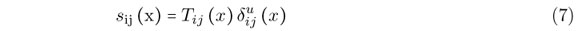The relative displacement can be formulated by help of axial displacement u and deflection vM of beams as followswherethe deflection of beam i caused by bending. Substituting Eq. (8) into Eq. (7) results in the following equation for the shear forceAnd the longitudinal shear stiffness matrix is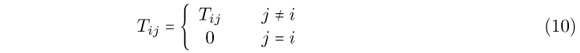The next type of coupling is vertical coupling. This type of coupling is of great importance when the superstructure is weakly supported. This condition is well described by Bleich . The interaction between beams i and j is described with distributed vertical springs in Fig. 7. Vertical coupling force pij depends on vertical coupling stiffness kij and relative deflection, which is the difference between beam deflections vi and vj. Hence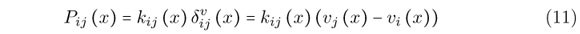Using the beam theory, the relations between the internal forces and displacements are defined assuming that the material follows Hooke's law. If axial displacement ui and deflectionvare known for beam i, then bending moment Mi and axial forces Ni are, see Crisfieldwhere parameters EAi and EIi are the axial stiffness and the bending stiffness of beam i with respect to the reference axis and EXi is the value which modifies the internal forces if the reference line differs from the centroid of the cross-section. Matrices EAi, EIi and EXi are diagonal.

2.3 Summary of equations to be solved

The following equations are to be solved in order to assess bending response of a ship using coupled beam method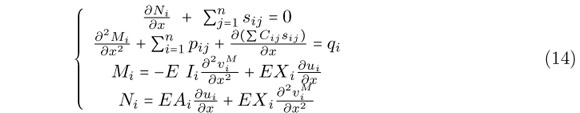Eliminating Mi and Ni in above equations, result in the following equationsThe unknowns in Eqs. (15) and (16) are axial deflection ui and transverse deflection induced by bending.

2.4 Characteristics of coupled beam method presented by Naar et al.

Naar et al. presented an approach, based on the coupled beam method, for estimation of bending response of ships having long superstructures. They assumed that the longitudinal shear force and transverse (vertical) force are equal to zero at both ends of superstructure. With such an assumption, shape functions are defined along the ship's hull and superstructure. In case of a ship having a short superstructure, the values of longitudinal shear force and vertical transverse force are not equal to zero at both ends of superstructure. Therefore, some modifications are necessary to be performed within the approach developed by Naar et al. in order to make it applicable to other types of ships having superstructures of any lengths. This is the main reason behind works presented in this manuscript.

3 EXTENDED FORMULATION

3.1 General

In order to resolve existing inabilities in the approach presented by Naar et al. regarding bending response analysis of ships with short superstructures, a new method is provided in this paper. The adopted concept for discretisation of ship structure into different beam and spring elements is shown schematically in the Fig. 8. As can be seen in Fig. 8, both hull and superstructure are modelled as beams consisting of a number of beam elements. In the connecting region between hull and superstructure, the nodes are so located to have the same abscissa. The beam elements are of three-node type, having a total number of six degrees of freedom, so that the variations in the axial force can be considered. The reference line is considered at the deck level.

The beams representing hull and superstructure are connected to each other using the so-called 'spring box elements'. The stiffness matrix of these spring box elements is derived using equilibrium conditions. Any spring box elements consist of 9 transverse springs and also 9 shear springs, Fig. 9. The transverse springs and shear springs are simulating respectively vertical forces and shear forces acting between the two beam elements, one inside the hull and the other one inside the superstructure.

The governing equations to be solved were summarised in Section 2.3. The boundary conditions are of free-free type. The Galerkin method  is adopted in order to solve the set of equations. Using the Galerkin method, the finite element equations are formulated. The length of the ship is divided into m intervals. The first node is chosen at the after perpendicular position, while the last node is placed at the forward perpendicular. Each of the intervals includes three nodes.andare approximate solutions for the ui andfunctions in i-th interval. These approximate solutions are considered as linear combination of the corresponding nodal deflections in the following mannerDegrees of freedom in above equations are as follows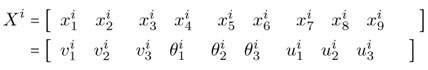where,andare nodal angular displacement and nodal displacement of i-th beam in normal direction respectively.

The shape functions Nj(x) are obtained as followsThe residuals would be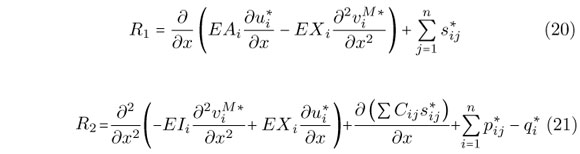Based on the Galerkin method, in order to have minimum error, the functionsandhave to satisfy the following equations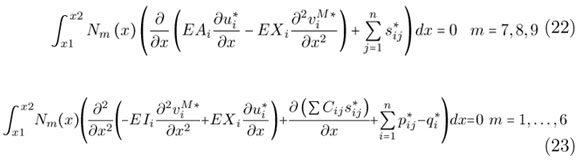Integrating Eqs. (22) and (23) by part results inThe stiffness matrices for the beam elements and spring box elements can be easily obtained using Eq. (24). More details on these are given in the next sections.

3.2 Assembling algorithm

After derivation of stiffness matrices for hull beam elements, superstructure beam elements and also spring box elements, the global stiffness matrix of the whole ship structure is assembled. Since the shear force and bending moment are zero at both ends of the ship, in order to solve the finite element equations, singular points should be eliminated so that rigid body motion of the ship is prevented. The resulting set of finite element equations is then solved. Figure 10 shows the flow of steps from beginning towards the solution.

3.3 Stiffness matrix of the beam elements

Based on the Eq. (24), the stiffness matrix elements for i-th beam element having nodes 1, 2 and 3 with degrees of freedom u, vM and θ per each node would take the following form∈ K

1 < m, j < 9

where Ki is the stiffness matrix of i-th beam.

Since the sectional properties of a ship are generally variable along its length, it would be more accurate if the sectional properties of the beam elements can also vary along their length. Therefore, the quantities EAi, EXi and EIi are defined in the new formulation as follows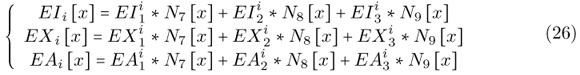where Ii, Ai and Xi are second moment of inertia, cross-sectional area and first sectional moment of area at node number i of the beam element, which can be calculated by the following equationsUsing above definitions, the stiffness matrix takes the following formThe vector of degrees of freedom for i-th beam element isand the matrices KEAj, KEXj and KEAj are explained in the appendix.

3.4 Stiffness matrix of the spring box elements

Spring box elements are used in order to simulate the connection between beam elements of the hull and beam elements of the superstructure. The forces acting on the beam elements from the spring box elements areand, which are respectively representing axial force per unit length, transverse force per unit length and bending moment per unit length.

Any one of spring box elements has 6 nodes, a total of 18 degrees of freedom, shear stiffness and transverse vertical stiffness. The shear stiffness matrix and transverse vertical matrix areand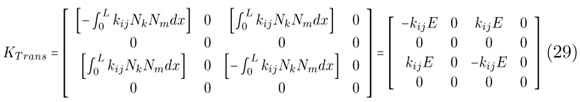Matrices A, B, D and E are given in the appendix.

3.5 Elimination of singular points of solution of finite element equations

The global stiffness matrix of the whole ship is singular, because there are not enough support constraints to prevent its rigid body motion. In these cases, Singular Value Decomposition (SVD) offers a better solution in many respects. All matrices have a unique decomposition as multiplication of three matrices, a square orthogonal matrix, a diagonal matrix and a square orthogonal matrix. Therefore, K, the global stiffness matrix of system, can be written as bellowwhere U and V are square real and orthogonal. diag is a diagonal matrix that contains the singular values. In terms of U, V, and diag, the system is readily solved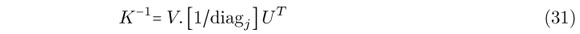U and V being orthogonal means that their transposes are also their inverses. The inverse of a diagonal matrix is just the reciprocal of the diagonal elements. The problem exists when the system consists of one or more singular values (diagj). This means that diagj is very small compared with the largest diagonal matrix element. It follows that (1/diagj) is a very large number, which distorts the numerical solution, sending it off to infinity along a direction which is spurious. A good approximation is to throw these spurious directions away completely by setting (1/diagj) for the offending singular values to zero. The vectorwhere F and Xs are vector of external forces and nodal displacement vector of system respectively.

3.6 Implementation

Newly extended formulation was implemented in a code that was written in MATLAB environment. The code creates the set of finite element equations for the whole ship structure considering the couplings among beam elements of the hull and superstructure. The inputs of the code are typically coordinates of the nodes, beam element connectivity matrix, spring connectivity matrix and also vector of the loads. The outputs of the code consist of deflection components at the nodes, internal forces and moments at the nodes and also longitudinal stress at the nodes.

4 VALIDATION

Mackney and Rose  tested a simple ship model with the scale of 1/60 in a four-point bending mechanism. The model had a length of 2 m, a breadth of 0.25 m, a hull height of 0.167 m and a superstructure height of 0.117 m. The experimentally obtained deflections for the model are shown in Fig. 11 with the marked points. The numerical results using the developed code are also shown in the Fig. 11 with the solid line. As can be seen, a very good correlation exists among the results.

5 NUMERICAL EXPERIMENTS AND DISCUSSIONS

Two models are created numerically and their bending responses are assessed using the developed code. In order to examine validity of the results obtained based on the extended formulation; the same models are analysed using ANSYS finite element code . Different paths are considered along the models where longitudinal stress distributions obtained based on the present formulation and ANSYS are compared with each at them. All the forces applied on the models are self-balanced. That means there are no forces acting on the imaginary support considered for the model. This support could be placed at any location on the natural axis of the section. Herein, in order to prevent rigid body motion of the models, a point on the midlength of the hull, but located on the neutral axis of the section, is restrained against motion. Elements of SHELL63  type were used in order to discretise the models. SHELL63 element has both bending and membrane capabilities. Both in-plane and normal loads are permitted. The element has six degrees of freedom at each node: translations in the nodal x, y, and z directions and rotations about the nodal x, y, and z axes. Stress stiffening and large deflection capabilities are included.

5.1 Case study 1: box girder model with a superstructure shorter than the hull

Model number 1 is a box girder model in which the superstructure has a length smaller than that of the hull. Geometrical specifications of the model are shown in Fig. 12(a). Loading distribution for the model is also given in Fig. 12(b). In order to investigate longitudinal stress distributions for the model, 6 equidistant paths are considered between both ends of the superstructure (Fig. 12(a)).Figures 13 to 18 show the comparisons between the longitudinal stress distributions obtained using the extended formulation and ANSYS for different paths. Shear force and bending moment are equal to zero at both ends of superstructure. That is why it is realised that the proposed method approximates well the longitudinal stress distribution at both ends of the superstructure, Figs. 13 and 18 . The reason behind some existing errors between the results is mainly due to structural discontinuities at both ends of the superstructure. In real ship structures, a gradual transition in the form of curved brackets is considered at the hull-superstructure connections. Thus, the amount of stress concentration at the hull-superstructure is reduced reasonably to a lower level. The presented formulation has sufficient accuracy for the preliminary assessment of general strength of ship structures. However, the stress concentrations existing at the corners of hull-superstructure connections or around the side openings may be compensated using simple empirical equations that can be implemented in the present extended formulation. This remains as a future work.

Figure 14, 15, 16 and 17 also demonstrate this fact that the superstructure is contributing to the bending strength of the ship's hull. Again good correlations are observed among the results of the developed code and ANSYS.

5.2 Case study 2: box girder model with a long superstructure

In this case, bending behaviour of a model in which both hull and superstructure are of the same length is investigated. Geometrical specifications of the model are given in Fig. 19. Loading case is the same as that applied for the model number 1, Fig. 12(b). Six equidistant paths are considered along the ship. The longitudinal stress distributions obtained using ANSYS software are compared with those obtained using the proposed formulation for two typical paths in Figs. 20 and 21. As can be seen, a good agreement exists between the results of both methods.

6 CONCLUSIONS

This paper was aimed at investigation bending response of the ships considering the contribution of the superstructure. The coupled beam method developed by Naar et al. was further extended in a way that the resulting formulation could be capable of analysing the elastic bending response of the ships having superstructures of any lengths. The new extended formulation was coded in MATLAB environment and then it was validated against some available experimental data. Furthermore, some new cases were generated and their behaviours were examined using the extended formulation and ANSYS software. The results of the extended formulation for the numerical models were then compared with those results obtained using the finite element method and relatively good correlations were observed among them.

The extended formulation of the coupled beam method is so much effective in terms of CPU time and accuracy that can be easily implemented in the algorithms for assessment of the longitudinal bending strength of ship structures, considering the contribution coming from superstructures.

Improvements of the extended formulation in order to enable it to assess the stresses at discontinuities more accurately, such as hull-superstructure connections and side openings, are recommended to be performed as future works.

In revised form 06 Apr 2011

APPENDIX•  C. Andreau and L. Gillet. Structural design improvements of passenger ships. In IMAS 88, The Design and Development of Passenger Ships, 1988.
•  J. Andric, V. Zanic, and M. Grgic. Superstructure deck effectiveness in longitudinal strength of livestock carrier. In The 17th Symposium on Theory and Practice of Shipbuilding, SORTA 2006, Rijeka, Croatia, 2006.
•  H.H. Bleich. Non-linear distribution of bending stresses due to distortion of cross-section. J Appl Mech, 29:95104, 1952.
•  J. Bruhn. The stress at the discontinuities in a ship's structure. Trans RINA, pages 5763, 1899.
•  J.B. Caldwell. The effect of superstructure on the longitudinal strength of ships. Trans RINA, 99(4):664681, 1957.
•  J.C. Chapman. The interaction between a ship's hull and long superstructure. Trans RINA, 99(4):618633, 1957.
•  L. Crawford. Theory of long ship's superstructure. Trans SNAME, 58:693732, 1950.
•  M.A. Crisfield. Non-linear finite element analysis of solids and structures, volume 1. John Willey & Sons, West Sussex, England, 1991.
•  J.G. de Oliveira. Hull-deck interaction. In J.H. Evands, editor, Ship structural design concepts, second cycle, pages 160278, USA, 1983. Cornell Maritime Press.
•  J. Fransman. The influence of passenger ship superstructures on the response of the hull girder. Trans RINA, 131:5771, 1988.
•  M. Heder and A. Ulfvarson. Hull beam behavior of passenger ships. Marine structure, 4:1734, 1991.
•  S.E. Heggelund and T. Moan. Analysis of global loads effects in catamarans. Journal of Ship Research, 46(2):8191, 2002.
•  W. Hovgaard. A new theory of the distribution of shearing stresses in riveted and welded connection and its application to discontinuities in the structure of a ship. Trans RINA, pages 5259, 1931.
•  W. Hovgaard. The stress distribution in longitudinal welds and adjointing structures. J Math Phys, 13:195200, 1934.
•  D.V. Hutton. Fundamentals of finite element analysis McGraw Hill, 2004.
•  A.J. Johnson. Stresses in deckhouse and superstructure. Trans RINA, 99(4):634663, 1957.
•  J.F. King. On large deckhouse. Trans RINA, pages 148161, 1913.
•  M.D.A. Mackney and C.T.F. Rose. Preliminary ship design using one-and two-dimensional models. Marine technology, 36(2):102111, 1999.
•  J.D. McVee. A finite element study of hull-deckhouse interaction. Computers & Structures, 12:371393, 1980.
•  G.C. Mitchell. Analysis of structural interaction between a ship's hull and deckhouse. Trans RINA, 120:121134, 1978.
•  H. Naar, P. Varsta, and P. Kujala. A theory of coupled beams for strength assessment of passenger ships. Marine structures, 17:590611, 2004.
•  J.R. Paulling and H.G. Payer. Hull-deckhouse interaction by finite element calculations. Trans SNAME, 76:281296, 1968.
•  Swanson Analysis Systems Inc., Houston. ANSYS User's Manual (Version 7.1), 2003.
•  K. Terazava and J. Yagi. Stress distribution in deckhouse and superstructure. The Society of Naval Architects of Japan, 60th Anniversary Series, 9:51150, 1964.
•  J. Vasta. Structural tests on the libery ship S.S. Philip Schuyer. Trans SNAME, 55:391396, 1947.
•  J. Vasta. Structural tests on the passenger ship S.S. President Wilson Interaction between superstructure and main hull girder. Trans SNAME, 57:253306, 1949.
•  V. Zanic and T. Jancijev. Structural design and analysis of night ferry Amorella with 2200 passengers and 600 cars. Technical report, BRODOSPLIT Shipyard, University of Zagreb, 1986.
•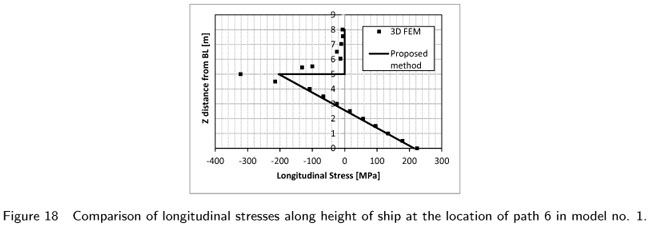• *
Author email:

# Publication Dates

• Publication in this collection
19 Dec 2011
• Date of issue
Sept 2011